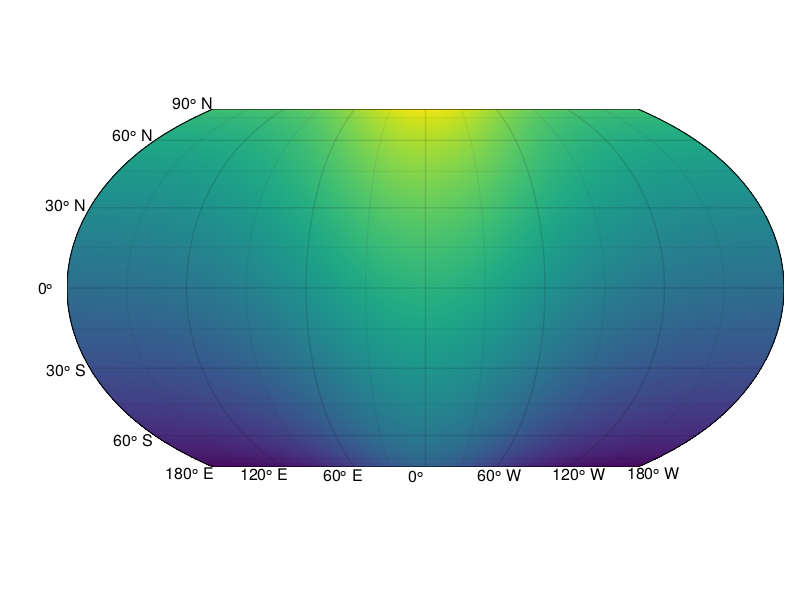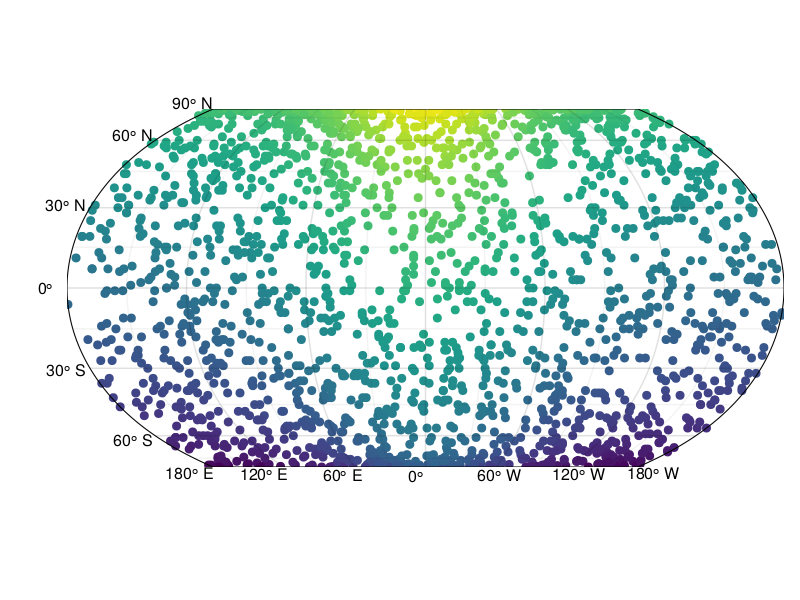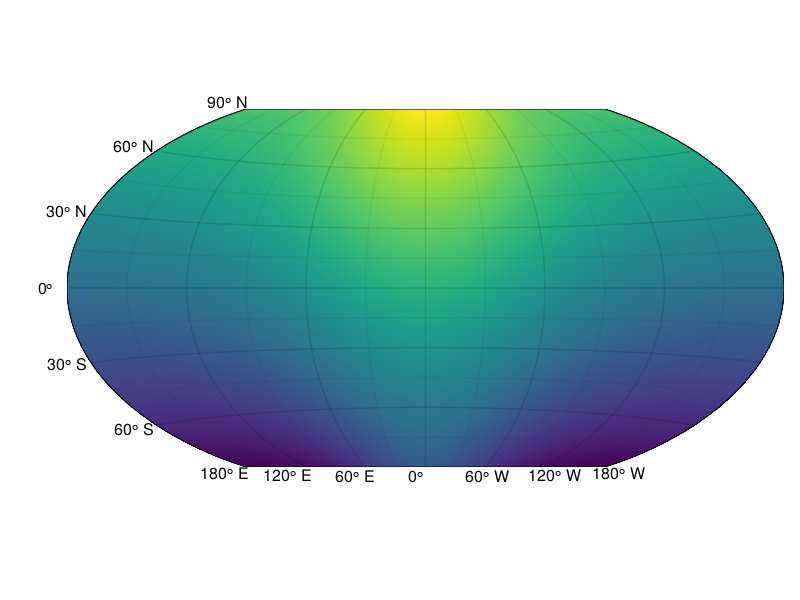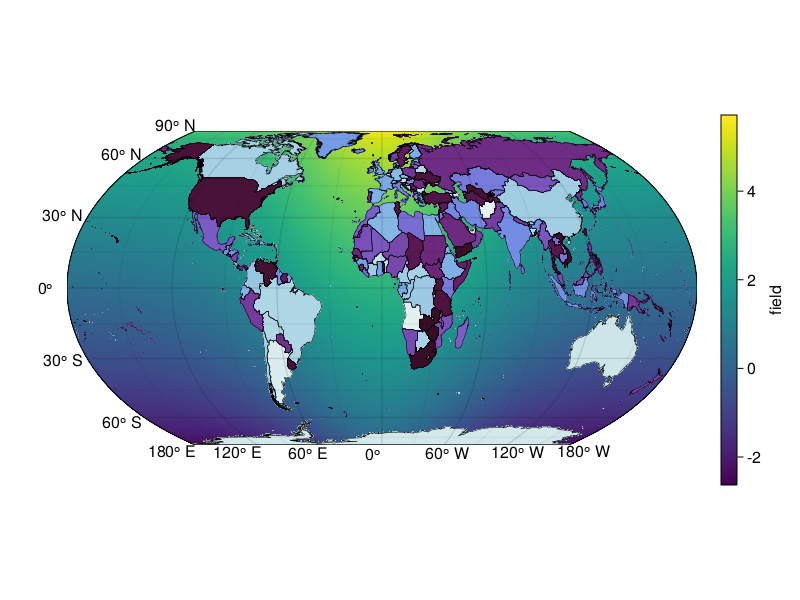# GeoMakie.jl

GeoMakie.jl is a Julia package for plotting geospatial data on a given map projection. It is based on the Makie.jl plotting ecosystem.

The package ClimateBase.jl builds upon GeoMakie.jl to create a seamless workflow between analyzing/manipulating climate data, and plotting them.

## Installation

This package is in development and may break, although we are currently working on a long-term stable interface.

You can install it from the REPL like so:

]add GeoMakie

## GeoAxis

Using GeoMakie.jl is straightforward, although it does assume basic knowledge of the Makie.jl ecosystem.

GeoMakie.jl provides an axis for plotting geospatial data, GeoAxis, and also the function geo2basic that converts an output of GeoJSON to a polygon appropriate for plotting. Both are showcased in the examples below.

GeoMakie.GeoAxisFunction
GeoAxis(fig_or_scene; kwargs...) → ax::Axis

Create a modified Axis of the Makie.jl ecosystem. All Makie.jl plotting functions work directly on GeoAxis, e.g., scatter!(ax, x, y). You can pass any keyword which Axis accepts, and manipulate it just like a regular Axis.

This is because it is a regular Axis, using the interface you are already familiar with, functions like xlims! and attributes like ax.xticks, etc. just work.

GeoAxis is appropriate for geospatial plotting because it automatically transforms all plotted data, given a user-defined map projection. See keyword arguments below and examples in the online documentation. Longitude and latitude values in GeoMakie.jl are always assumed to be in degrees.

In order to automatically adjust the limits to your data, you can call datalims!(ax) on any GeoAxis.

In the call signature, fig_or_scene can be a standard figure location, e.g., fig[1,1] as given in Axis. The keyword arguments decide the geospatial projection.

Keyword arguments

• source = "+proj=longlat +datum=WGS84", dest = "+proj=eqearth": These two keywords configure the map projection to be used for the given field using Proj.jl. See also online the section Changing central longitude for data that may not span the (expected by default) longitude range from -180 to 180.
• transformation = Proj.Transformation(source, dest, always_xy=true): Instead of source, dest, you can directly use the Proj.jl package to define the projection.
• lonlims = (-180, 180): The limits for longitude (x-axis). For automatic determination, pass lonlims=automatic.
• latlims = (-90, 90): The limits for latitude (y-axis). For automatic determination, pass latlims=automatic.
• coastlines = false: Draw the coastlines of the world, from the Natural Earth dataset.
• coastline_attributes = (;): Attributes that get passed to the lines call drawing the coastline.
• line_density = 1000: The number of points sampled per grid line. Do not set this higher than 10,000 for performance and file size reasons..
• remove_overlapping_ticks = true: Remove ticks which could overlap each other. X-axis (longitude) ticks take priority over Y-axis (latitude) ticks.

Example

using GeoMakie
fig = Figure()
ax = GeoAxis(fig[1,1]; coastlines = true)
image!(ax, -180..180, -90..90, rotr90(GeoMakie.earth()); interpolate = false)
el = scatter!(rand(-180:180, 5), rand(-90:90, 5); color = rand(RGBf, 5))
fig

source
GeoMakie.geo2basicFunction
geo2basic(obj::GeoInterface)

Converts any GeoInterface object to the equivalent GeometryBasics, which can be plotted by Makie.

source

## Gotchas

When plotting a projection which has a limited domain (in either longitude or latitude), if your limits are not inside that domain, the axis will appear blank. To fix this, simply correct the limits - you can even do it on the fly, using the xlims!(ax, low, high) or ylims!(ax, low, high) functions.

## Examples

### Surface example

using GeoMakie, CairoMakie

lons = -180:180
lats = -90:90
field = [exp(cosd(l)) + 3(y/90) for l in lons, y in lats]

fig = Figure()
ax = GeoAxis(fig[1,1])
surface!(ax, lons, lats, field; shading = false)
fig### Scatter example

using GeoMakie, CairoMakie

lons = -180:180
lats = -90:90
slons = rand(lons, 2000)
slats = rand(lats, 2000)
sfield = [exp(cosd(l)) + 3(y/90) for (l,y) in zip(slons, slats)]

fig = Figure()
ax = GeoAxis(fig[1,1])
scatter!(slons, slats; color = sfield)
fig### Map projections

The default projection is given by the arguments source = "+proj=longlat +datum=WGS84", dest = "+proj=eqearth", so that if a different one is needed, for example a wintri projection one can do it as follows:

using GeoMakie, CairoMakie

lons = -180:180
lats = -90:90
field = [exp(cosd(l)) + 3(y/90) for l in lons, y in lats]

fig = Figure()
ax = GeoAxis(fig[1,1]; dest = "+proj=wintri")
surface!(ax, lons, lats, field; shading = false)
fig### Changing central longitude

Be careful! Each data point is transformed individually. However, when using surface or contour plots this can lead to errors when the longitudinal dimension "wraps" around the planet.

E.g., if the data have the dimensions

lons = 0.5:359.5
lats = -90:90
field = [exp(cosd(l)) + 3(y/90) for l in lons, y in lats];
360×181 Matrix{Float64}:
-0.281822  -0.248488  -0.215155  …  5.61818  5.65151  5.68484  5.71818
-0.282649  -0.249316  -0.215983     5.61735  5.65068  5.68402  5.71735
-0.284304  -0.250971  -0.217637     5.6157   5.64903  5.68236  5.7157
-0.286784  -0.25345   -0.220117     5.61322  5.64655  5.67988  5.71322
-0.290085  -0.256751  -0.223418     5.60992  5.64325  5.67658  5.70992
-0.294204  -0.260871  -0.227537  …  5.6058   5.63913  5.67246  5.7058
-0.299136  -0.265802  -0.232469     5.60086  5.6342   5.66753  5.70086
-0.304874  -0.271541  -0.238208     5.59513  5.62846  5.66179  5.69513
-0.311413  -0.278079  -0.244746     5.58859  5.62192  5.65525  5.68859
-0.318743  -0.28541   -0.252077     5.58126  5.61459  5.64792  5.68126
⋮                               ⋱                             ⋮
-0.311413  -0.278079  -0.244746     5.58859  5.62192  5.65525  5.68859
-0.304874  -0.271541  -0.238208     5.59513  5.62846  5.66179  5.69513
-0.299136  -0.265802  -0.232469     5.60086  5.6342   5.66753  5.70086
-0.294204  -0.260871  -0.227537     5.6058   5.63913  5.67246  5.7058
-0.290085  -0.256751  -0.223418  …  5.60992  5.64325  5.67658  5.70992
-0.286784  -0.25345   -0.220117     5.61322  5.64655  5.67988  5.71322
-0.284304  -0.250971  -0.217637     5.6157   5.64903  5.68236  5.7157
-0.282649  -0.249316  -0.215983     5.61735  5.65068  5.68402  5.71735
-0.281822  -0.248488  -0.215155     5.61818  5.65151  5.68484  5.71818

a surface! plot with the default arguments will lead to artifacts if the data along longitude 179 and 180 have significantly different values. To fix this, there are two approaches: (1) to change the central longitude of the map transformation, by changing the projection destination used like so:

ax = GeoAxis(fig[1,1]; dest = "+proj=eqearth +lon_0=180")

or (2), circshift your data appropriately so that the central longitude you want coincides with the center of the longitude dimension of the data.

using GeoMakie, CairoMakie

# First, make a surface plot
lons = -180:180
lats = -90:90
field = [exp(cosd(l)) + 3(y/90) for l in lons, y in lats]

fig = Figure()
ax = GeoAxis(fig[1,1])
sf = surface!(ax, lons, lats, field; shading = false)
cb1 = Colorbar(fig[1,2], sf; label = "field", height = Relative(0.65))

using GeoMakie.GeoJSON

n = length(GeoInterface.features(countries))
hm = poly!(ax, countries; color= 1:n, colormap = :dense,
strokecolor = :black, strokewidth = 0.5, overdraw = true,
)

# cb2 = Colorbar(fig[1,3], hm; label = "countries index", height = Relative(0.65))

fig## Gotchas

With CairoMakie, we recommend that you use image!(ga, ...) or heatmap!(ga, ...) to plot images or scalar fields into ga::GeoAxis.

However, with GLMakie, which is much faster, these methods do not work; if you have used them, you will see an empty axis. If you want to plot an image img, you can use a surface in the following way: surface!(ga, lonmin..lonmax, latmin..latmax, ones(size(img)...); color = img, shading = false).

To plot a scalar field, simply use surface!(ga, lonmin..lonmax, latmin..latmax, field). The .. notation denotes an interval which Makie will automatically sample from to obtain the x and y points for the surface.